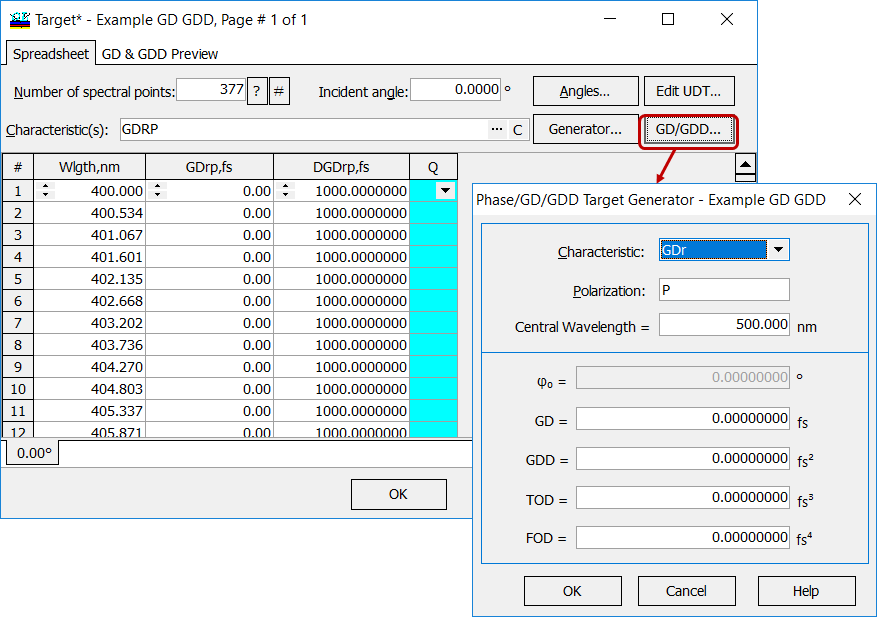# Phase/GD/GDD Targets

 Phase/GD/GDD targets are necessary for designing dispersive mirrors and other coatings for ultrafast applications. Phase/GD and GDD targets can be specified with the help of Target Generator, Grid Generator or a hot key F6. OptiLayer has also a special Phase/GD/GDD Target Generator dialog that can be used for quick computations of Phase, GD, and GDD wavelength dependencies on the basis of initial values often used by specialists in ultrafast optics. In the dialog it is possible to specify initial phase value (only for phase targets), GD value (only for phase and GD targets), GDD, TOD and FOD values at the Central wavelength. Target characteristics are evaluated according to formulas ($$\omega_0$$ is the frequency corresponding to the Central Wavelength):Phase Targets $$(\varphi r, \varphi t)$$: $\varphi (\omega)=\varphi_0-GD\cdot (\omega-\omega_0)-1/2 \cdot GDD\cdot (\omega-\omega_0)^2-1/6\cdot TOD\cdot (\omega-\omega_0)^3-1/24\cdot FOD\cdot (\omega-\omega_0)^4$ GD Target (GDr, GDt): $GD (\omega)=GD+1/2 \cdot TOD\cdot (\omega-\omega_0)^2+1/6\cdot FOD\cdot (\omega-\omega_0)^3$ GDD Target (GDDr, GDDt): $GDD (\omega)=GDD+TOD\cdot (\omega-\omega_0)+1/2\cdot FOD\cdot (\omega-\omega_0)^2$

### Look our video examples

Look our video examples at YouTube

OptiLayer videos are available here:
Overview of Design/Analysis options of OptiLayer and overview of Characterization/Reverse Engineering options.

The videos were presented at the joint Agilent/OptiLayer webinar.